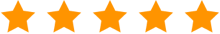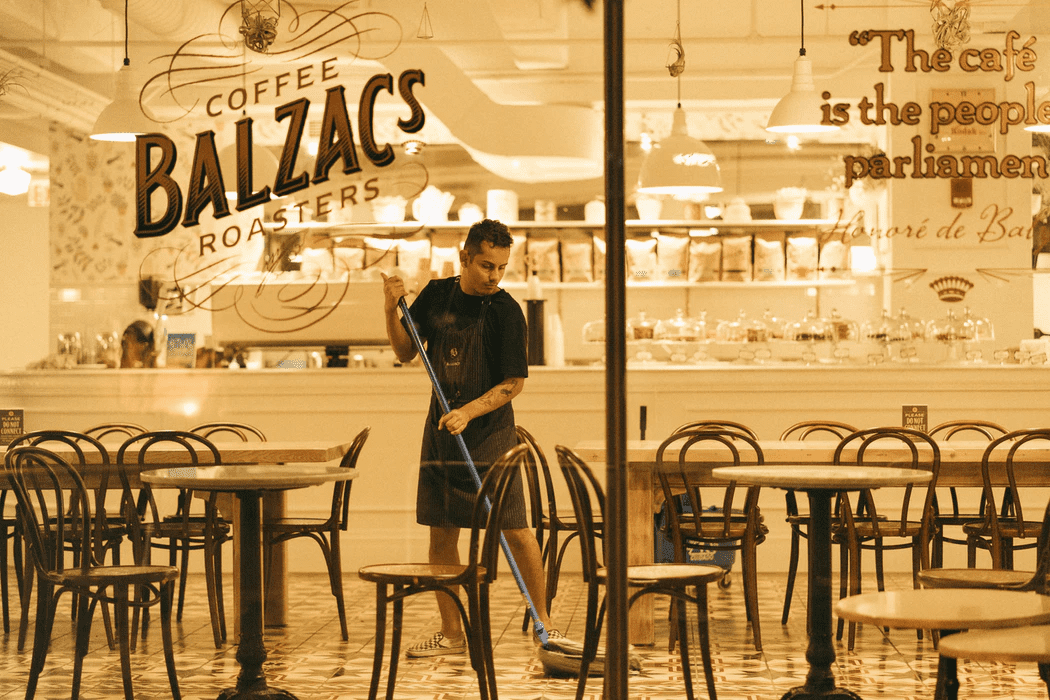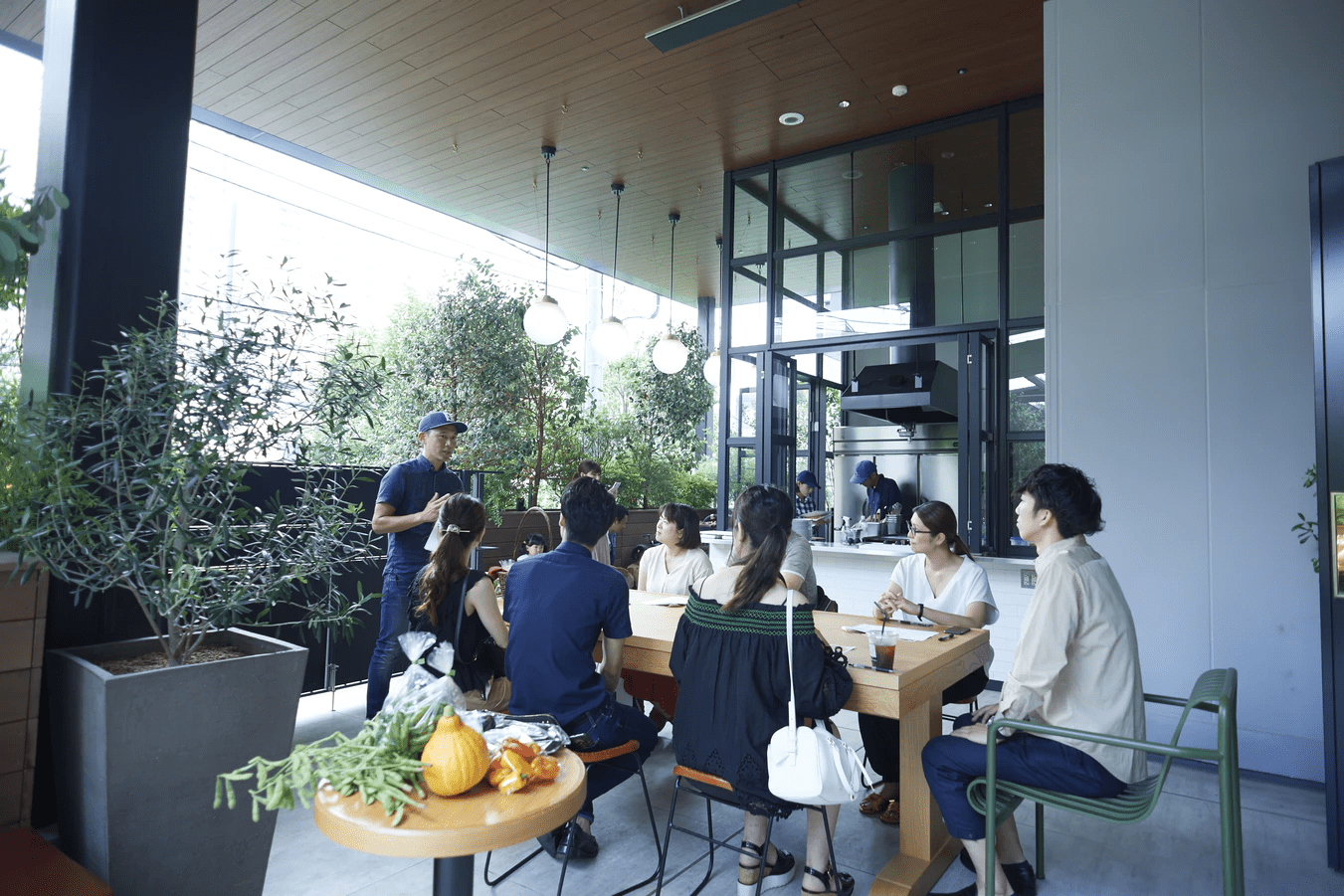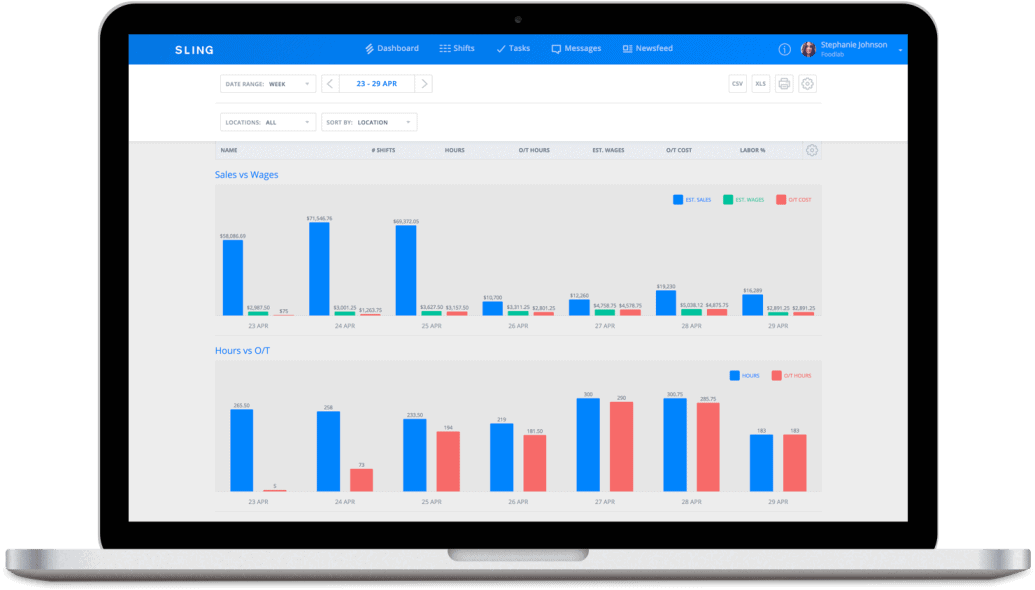"Sling allows businesses to schedule smarter instead of harder." - Bradley Knebel

## How To Calculate And Control Restaurant Labor CostCalculating and controlling restaurant labor costs is essential for the success of your business. If you’re not familiar with the key metrics that result in labor cost and labor cost percentage, it’s very difficult to get control of your bottom line.

In this article, the restaurant-management experts at Sling give you four easy steps to calculate your restaurant labor cost and then provide suggestions for controlling these numbers.

## A Bit About Prime CostFor restaurants, prime cost is the combination of two expenses:

• Food and beverage
• Labor

Every restaurant is different — and you may find a prime cost that works better for you — but, as a general rule, prime cost should fall between 55 and 60 percent of weekly sales. Of that 55-60 percent, labor costs should make up 15-17 percent of weekly sales.

Now, we’ll delve a bit deeper into calculating restaurant labor costs so you can discover ways to improve your bottom line.

## How To Calculate Restaurant Labor CostsThe easiest way to calculate restaurant labor costs is to examine your numbers from a previous shift, day, week, or (better yet) full month of activity.

Armed with this data, you can then begin to estimate labor costs and control your employee schedule to keep expenses low (we’ll talk about this at the end of the article).

Here’s how to calculate restaurant labor cost in four easy steps:

1. Choose a time period
2. Determine the number of guests for that time period
3. Determine the minimum number of staff needed to serve guests
4. Gather wage data and use it to figure out restaurant expenses (a.k.a. labor cost for the time in question)

To help you understand this process, we’ve set up a hypothetical restaurant in the next section.

We’ll walk through the numbers and equations for this hypothetical restaurant so you can learn how to apply the calculations to your own business.

### Hypothetical Restaurant Labor Cost

Beginning with step one — choose a time period — it’s often easier to start small and work up from there. So instead of trying to analyze a whole week of numbers, we’ll use two hours during the lunch shift on our busiest day.

In step two — determine the number of guests — we go back through our point of sale data to find the number of guests who ate during the lunch shift on our busiest day.

In our hypothetical restaurant example, we’ll use 50 guests in two hours.

Now that we know the number of guests, we can move to step three — determine the minimum number of staff needed to serve those 50 guests.Keep in mind that you may have been short-staffed during the time in question, so set the number of staff needed at your ideal level. That way, you can use these numbers to project your labor costs into the future.

For our hypothetical restaurant, we’ll use the following metrics:

• 2 hosts
• 5 servers
• 3 cooks

When we know how many team members we need, we can accurately determine labor cost for those two hours in step four.

In our hypothetical restaurant, hosts are paid \$12/hour, servers are paid \$10/hour, and cooks are paid \$15/hour.

With that information, we can multiply each employee by the hours they work (two) and the wage they are paid.

Here’s what that looks like for this example:

2 hosts x (2 hours worked x \$12/hour) = \$48

5 servers x (2 hours worked x \$10/hour) = \$100

3 cooks x (2 hours worked x \$15/hour) = \$90

Now all we have to do is add those numbers together to find our total restaurant labor cost.

Restaurant Labor Cost = The Sum Of All Wages Paid During Two Hours

Restaurant Labor Cost = \$48 + \$100 + \$90

Restaurant Labor Cost = \$238

So \$238 is the labor cost for our restaurant’s busiest lunch shift. But that is just a number that doesn’t really tell us anything other than what we spent on wages. To really get a handle on our spending, we now need to calculate labor cost percentage.

## How To Calculate Restaurant Labor Cost PercentageUsing some of the data you’ve already collected, you can calculate restaurant labor cost percentage in three easy steps:

1. Determine the average check
2. Calculate total income
3. Plug and chug to figure out how your labor costs relate to your prime cost (a.k.a. labor cost percentage)

Again, we’ll walk through our hypothetical restaurant’s numbers as an example.

### Hypothetical Restaurant Labor Cost Percentage

We’ll start calculating labor cost percentage at step one by determining the average check for the 50 guests during the two-hour lunch shift. For our hypothetical restaurant, we’ll set the average check at \$25.

Moving on to step two, we can calculate total income by multiplying the average check by the number of guests that dined.

Total Income = Average Check x Number Of Guests

Total Income = \$25 x 50

Total Income = \$1250

That’s how much money our hypothetical restaurant brought in (on average) during the two-hour period in question.

Finally, in step three, we can use all this data to calculate our hypothetical restaurant labor cost percentage.

Here’s the equation:

Restaurant Labor Cost Percentage = Restaurant Labor Cost / Total Income

Restaurant Labor Cost Percentage = \$238 / \$1250

Restaurant Labor Cost Percentage = 0.19

We talked about prime cost (food, beverage, and labor cost combined) at the beginning of this article and how labor costs should be 15-17 percent of that number. The 0.19 (or 19 percent) we calculated above tells that our labor costs are a bit high (greater than 17 percent).

Now that we know what our labor costs are, we can take steps to control them.

## How To Control Restaurant Labor CostOnce you’ve identified the values of your key metrics (prime cost, labor costs, and labor cost percentage), you can begin to adjust the way your business operates to increase profits.

Here are some of the best ways to do that:

1. Create an appropriate salary structure
2. Control attrition
4. Invest in hiring
5. Assemble a diverse team
7. Improve efficiency
8. Track time and attendance

## Manage Labor Cost With Scheduling SoftwareThe best way to manage and lower your labor costs is to use a suite of software tools like Sling.

Sling gives you incredible control over your schedule so you can quickly and easily create schedules one month, two months, even six months or more in advance.

Sling even lets you optimize labor costs by setting wages per employee or position so you can see how much each shift will cost you.